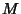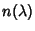## Milnor's Theorem

If a Compact Manifoldhas Nonnegative Ricci Curvature, then its Fundamental Group has at most Polynomial growth. On the other hand, ifhas Negative curvature, then its Fundamental Group has exponential growth in the sense thatgrows exponentially, whereis (essentially) the number of different words'' of lengthwhich can be made in the Fundamental Group.

References

Chavel, I. Riemannian Geometry: A Modern Introduction. New York: Cambridge University Press, 1994.